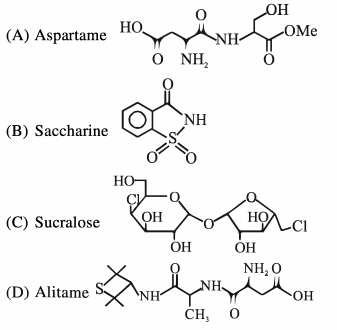# A chemist has 4 samples of artificial sweetener

Question:

A chemist has 4 samples of artificial sweetener A, B, C and D. To identify these samples, he performed certain experiments and noted the following observations :

(i) A and D both form blue-violet colour with ninhydrin.

(ii) Lassaigne extract of $\mathrm{C}$ gives positive $\mathrm{AgNO}_{3}$ test and negative $\mathrm{Fe}_{4}\left[\mathrm{Fe}(\mathrm{CN})_{6}\right]_{3}$ test.

(iii)Lassaigne extract of $B$ and $D$ gives positive sodium nitroprusside test

Based on these observations which option is correct ?

1. A : Aspartame ; B : Saccharin ;

C : Sucralose ; D ; Alitame

2. A : Alitame ; B : Saccharin ;

C : Aspartame ; D ; Sucralose

3. A : Saccharin ; B : Alitame ;

C : Sucralose ; D ; Aspartame

4. A : Aspartame ; B : Alitame ;

C : Saccharin ; D ; Sucralose

Correct Option: 1

Solution:

(i) Blue voilet color with Ninhydrine $\rightarrow$ amino acid derivative. So it cannot be saccharide or sucralose.

(ii) Lassaigne extract give +ve test with $\mathrm{AgNO}_{3} . \mathrm{So} \mathrm{Cl}$ is present, -ve test with $\mathrm{Fe}_{4}\left[\mathrm{Fe}(\mathrm{CN})_{6}\right]_{3}$ means $\mathrm{N}$ is absent. So it can't be Aspartame or Saccharine or Alitame, so $C$ is sucralose.

(iii) Lassaigne solution of B and D given +ve sodium nitroprusside test, so it is having $S$, so it is Saccharine and Alitame.